# Mean State

Period Mean (original grids) [W/m2]
Model Period Mean (intersection) [W/m2]
Model Period Mean (complement) [W/m2]
Benchmark Period Mean (intersection) [W/m2]
Benchmark Period Mean (complement) [W/m2]
Bias [W/m2]
RMSE [W/m2]
Phase Shift [months]
Bias Score 
RMSE Score 
Seasonal Cycle Score 
Spatial Distribution Score 
Interannual Variability Score 
Overall Score 
Benchmark [-] 187.
CLM4 [-] 181. 181. 186. 206. -5.09 15.5 0.746 0.711 0.498 0.911 0.918 0.806 0.724
CLM4.5 [-] 181. 181. 186. 206. -4.81 15.3 0.744 0.717 0.500 0.914 0.931 0.806 0.728
CLM5 [-] 182. 182. 186. 206. -4.45 15.1 0.823 0.728 0.493 0.901 0.939 0.801 0.726
Period Mean (original grids) [W/m2]
Model Period Mean (intersection) [W/m2]
Model Period Mean (complement) [W/m2]
Benchmark Period Mean (intersection) [W/m2]
Benchmark Period Mean (complement) [W/m2]
Bias [W/m2]
RMSE [W/m2]
Phase Shift [months]
Bias Score 
RMSE Score 
Seasonal Cycle Score 
Spatial Distribution Score 
Interannual Variability Score 
Overall Score 
Benchmark [-] 198.
CLM4 [-] 193. 193. 198. 214. -4.63 14.4 0.711 0.704 0.517 0.923 0.982 0.857 0.750
CLM4.5 [-] 194. 194. 198. 214. -3.67 14.3 0.718 0.711 0.516 0.922 0.975 0.862 0.750
CLM5 [-] 195. 195. 198. 214. -2.80 14.2 0.767 0.714 0.516 0.906 0.964 0.852 0.745
Period Mean (original grids) [W/m2]
Model Period Mean (intersection) [W/m2]
Model Period Mean (complement) [W/m2]
Benchmark Period Mean (intersection) [W/m2]
Benchmark Period Mean (complement) [W/m2]
Bias [W/m2]
RMSE [W/m2]
Phase Shift [months]
Bias Score 
RMSE Score 
Seasonal Cycle Score 
Spatial Distribution Score 
Interannual Variability Score 
Overall Score 
Benchmark [-] 199.
CLM4 [-] 195. 195. 198. 219. -3.69 15.6 0.757 0.800 0.572 0.896 0.995 0.750 0.764
CLM4.5 [-] 196. 196. 198. 219. -3.23 15.5 0.742 0.797 0.573 0.899 0.994 0.751 0.765
CLM5 [-] 196. 196. 198. 219. -2.87 15.6 0.787 0.797 0.569 0.893 0.996 0.748 0.762
Period Mean (original grids) [W/m2]
Model Period Mean (intersection) [W/m2]
Model Period Mean (complement) [W/m2]
Benchmark Period Mean (intersection) [W/m2]
Benchmark Period Mean (complement) [W/m2]
Bias [W/m2]
RMSE [W/m2]
Phase Shift [months]
Bias Score 
RMSE Score 
Seasonal Cycle Score 
Spatial Distribution Score 
Interannual Variability Score 
Overall Score 
Benchmark [-] 70.7
CLM4 [-] 66.9 67.0 70.5 72.8 -3.66 20.9 0.355 0.906 0.741 0.976 0.997 0.804 0.861
CLM4.5 [-] 71.7 71.9 70.5 72.8 0.485 18.9 0.332 0.912 0.764 0.978 0.991 0.753 0.860
CLM5 [-] 73.1 73.3 70.5 72.8 1.86 18.4 0.331 0.906 0.772 0.978 0.996 0.741 0.861
Period Mean (original grids) [W/m2]
Model Period Mean (intersection) [W/m2]
Model Period Mean (complement) [W/m2]
Benchmark Period Mean (intersection) [W/m2]
Benchmark Period Mean (complement) [W/m2]
Bias [W/m2]
RMSE [W/m2]
Phase Shift [months]
Bias Score 
RMSE Score 
Seasonal Cycle Score 
Spatial Distribution Score 
Interannual Variability Score 
Overall Score 
Benchmark [-] 194.
CLM4 [-] 175. 175. 190. 207. -11.0 19.0 1.28 0.549 0.436 0.798 0.958 0.769 0.658
CLM4.5 [-] 175. 175. 190. 207. -10.9 19.0 1.24 0.552 0.436 0.803 0.956 0.769 0.659
CLM5 [-] 175. 175. 190. 207. -11.2 19.1 1.25 0.542 0.437 0.802 0.963 0.769 0.658
Period Mean (original grids) [W/m2]
Model Period Mean (intersection) [W/m2]
Model Period Mean (complement) [W/m2]
Benchmark Period Mean (intersection) [W/m2]
Benchmark Period Mean (complement) [W/m2]
Bias [W/m2]
RMSE [W/m2]
Phase Shift [months]
Bias Score 
RMSE Score 
Seasonal Cycle Score 
Spatial Distribution Score 
Interannual Variability Score 
Overall Score 
Benchmark [-] 97.8
CLM4 [-] 102. 102. 97.6 118. 4.49 15.6 0.227 0.914 0.819 0.985 0.998 0.691 0.871
CLM4.5 [-] 99.6 99.6 97.6 118. 2.33 16.3 0.232 0.916 0.808 0.985 0.998 0.694 0.868
CLM5 [-] 100. 100. 97.6 118. 2.99 15.5 0.231 0.921 0.817 0.985 0.995 0.687 0.870
Period Mean (original grids) [W/m2]
Model Period Mean (intersection) [W/m2]
Model Period Mean (complement) [W/m2]
Benchmark Period Mean (intersection) [W/m2]
Benchmark Period Mean (complement) [W/m2]
Bias [W/m2]
RMSE [W/m2]
Phase Shift [months]
Bias Score 
RMSE Score 
Seasonal Cycle Score 
Spatial Distribution Score 
Interannual Variability Score 
Overall Score 
Benchmark [-] 139.
CLM4 [-] 135. 134. 138. 149. -3.52 16.8 0.371 0.869 0.762 0.968 0.995 0.666 0.837
CLM4.5 [-] 134. 133. 138. 149. -4.24 16.5 0.344 0.866 0.767 0.971 0.997 0.660 0.838
CLM5 [-] 135. 135. 138. 149. -2.61 15.9 0.340 0.883 0.767 0.972 0.999 0.666 0.842
Period Mean (original grids) [W/m2]
Model Period Mean (intersection) [W/m2]
Model Period Mean (complement) [W/m2]
Benchmark Period Mean (intersection) [W/m2]
Benchmark Period Mean (complement) [W/m2]
Bias [W/m2]
RMSE [W/m2]
Phase Shift [months]
Bias Score 
RMSE Score 
Seasonal Cycle Score 
Spatial Distribution Score 
Interannual Variability Score 
Overall Score 
Benchmark [-] 181.
CLM4 [-] 173. 173. 179. 205. -4.55 18.4 0.346 0.765 0.610 0.972 0.971 0.730 0.776
CLM4.5 [-] 174. 173. 179. 205. -4.18 18.3 0.342 0.761 0.611 0.972 0.961 0.724 0.774
CLM5 [-] 174. 174. 179. 205. -3.72 18.1 0.320 0.770 0.610 0.974 0.938 0.722 0.771
Period Mean (original grids) [W/m2]
Model Period Mean (intersection) [W/m2]
Model Period Mean (complement) [W/m2]
Benchmark Period Mean (intersection) [W/m2]
Benchmark Period Mean (complement) [W/m2]
Bias [W/m2]
RMSE [W/m2]
Phase Shift [months]
Bias Score 
RMSE Score 
Seasonal Cycle Score 
Spatial Distribution Score 
Interannual Variability Score 
Overall Score 
Benchmark [-] 62.8
CLM4 [-] 59.4 59.8 63.2 54.4 -4.15 18.4 0.210 0.917 0.759 0.986 0.990 0.791 0.867
CLM4.5 [-] 62.0 62.3 63.2 54.4 -1.89 16.8 0.165 0.930 0.777 0.989 0.986 0.745 0.867
CLM5 [-] 64.7 65.0 63.2 54.4 0.684 15.6 0.199 0.931 0.792 0.987 0.990 0.748 0.873
Period Mean (original grids) [W/m2]
Model Period Mean (intersection) [W/m2]
Model Period Mean (complement) [W/m2]
Benchmark Period Mean (intersection) [W/m2]
Benchmark Period Mean (complement) [W/m2]
Bias [W/m2]
RMSE [W/m2]
Phase Shift [months]
Bias Score 
RMSE Score 
Seasonal Cycle Score 
Spatial Distribution Score 
Interannual Variability Score 
Overall Score 
Benchmark [-] 198.
CLM4 [-] 191. 191. 198. 197. -8.80 16.0 0.341 0.827 0.769 0.975 1.00 0.717 0.843
CLM4.5 [-] 191. 191. 198. 197. -8.85 16.1 0.334 0.824 0.769 0.975 1.00 0.718 0.842
CLM5 [-] 192. 192. 198. 197. -7.59 15.6 0.385 0.846 0.765 0.971 0.999 0.718 0.844
Period Mean (original grids) [W/m2]
Model Period Mean (intersection) [W/m2]
Model Period Mean (complement) [W/m2]
Benchmark Period Mean (intersection) [W/m2]
Benchmark Period Mean (complement) [W/m2]
Bias [W/m2]
RMSE [W/m2]
Phase Shift [months]
Bias Score 
RMSE Score 
Seasonal Cycle Score 
Spatial Distribution Score 
Interannual Variability Score 
Overall Score 
Benchmark [-] 120.
CLM4 [-] 112. 112. 118. 145. -3.16 14.9 0.242 0.916 0.824 0.984 0.998 0.683 0.872
CLM4.5 [-] 113. 113. 118. 145. -2.25 13.7 0.229 0.923 0.837 0.985 0.992 0.667 0.873
CLM5 [-] 113. 113. 118. 145. -2.30 13.6 0.227 0.921 0.839 0.985 0.990 0.660 0.872
Period Mean (original grids) [W/m2]
Model Period Mean (intersection) [W/m2]
Model Period Mean (complement) [W/m2]
Benchmark Period Mean (intersection) [W/m2]
Benchmark Period Mean (complement) [W/m2]
Bias [W/m2]
RMSE [W/m2]
Phase Shift [months]
Bias Score 
RMSE Score 
Seasonal Cycle Score 
Spatial Distribution Score 
Interannual Variability Score 
Overall Score 
Benchmark [-] 163.
CLM4 [-] 135. 135. 142. 173. -5.68 16.9 0.391 0.805 0.699 0.959 0.998 0.712 0.812
CLM4.5 [-] 136. 136. 142. 173. -5.10 16.2 0.383 0.815 0.707 0.960 0.998 0.719 0.817
CLM5 [-] 137. 137. 142. 173. -4.59 16.0 0.394 0.818 0.705 0.958 0.998 0.713 0.816
Period Mean (original grids) [W/m2]
Model Period Mean (intersection) [W/m2]
Model Period Mean (complement) [W/m2]
Benchmark Period Mean (intersection) [W/m2]
Benchmark Period Mean (complement) [W/m2]
Bias [W/m2]
RMSE [W/m2]
Phase Shift [months]
Bias Score 
RMSE Score 
Seasonal Cycle Score 
Spatial Distribution Score 
Interannual Variability Score 
Overall Score 
Benchmark [-] 177.
CLM4 [-] 160. 160. 175. 217. -13.9 18.9 0.288 0.686 0.770 0.979 0.961 0.667 0.805
CLM4.5 [-] 161. 161. 175. 217. -13.3 18.5 0.287 0.692 0.771 0.979 0.962 0.667 0.807
CLM5 [-] 162. 162. 175. 217. -12.0 18.0 0.296 0.708 0.763 0.978 0.960 0.674 0.808
Period Mean (original grids) [W/m2]
Model Period Mean (intersection) [W/m2]
Model Period Mean (complement) [W/m2]
Benchmark Period Mean (intersection) [W/m2]
Benchmark Period Mean (complement) [W/m2]
Bias [W/m2]
RMSE [W/m2]
Phase Shift [months]
Bias Score 
RMSE Score 
Seasonal Cycle Score 
Spatial Distribution Score 
Interannual Variability Score 
Overall Score 
Benchmark [-] 69.7
CLM4 [-] 67.6 67.5 69.6 71.1 -3.27 18.6 0.120 0.906 0.768 0.992 0.984 0.785 0.867
CLM4.5 [-] 70.6 70.5 69.6 71.1 -0.637 16.9 0.103 0.917 0.784 0.993 0.986 0.756 0.870
CLM5 [-] 70.7 70.6 69.6 71.1 -0.616 17.1 0.0938 0.915 0.784 0.994 0.981 0.747 0.867
Period Mean (original grids) [W/m2]
Model Period Mean (intersection) [W/m2]
Model Period Mean (complement) [W/m2]
Benchmark Period Mean (intersection) [W/m2]
Benchmark Period Mean (complement) [W/m2]
Bias [W/m2]
RMSE [W/m2]
Phase Shift [months]
Bias Score 
RMSE Score 
Seasonal Cycle Score 
Spatial Distribution Score 
Interannual Variability Score 
Overall Score 
Benchmark [-] 204.
CLM4 [-] 191. 192. 201. 219. -6.78 16.9 0.484 0.761 0.679 0.940 0.988 0.754 0.800
CLM4.5 [-] 191. 191. 201. 219. -7.27 17.0 0.495 0.762 0.678 0.940 0.987 0.757 0.800
CLM5 [-] 192. 192. 201. 219. -6.91 16.8 0.470 0.768 0.678 0.943 0.986 0.754 0.801
Period Mean (original grids) [W/m2]
Model Period Mean (intersection) [W/m2]
Model Period Mean (complement) [W/m2]
Benchmark Period Mean (intersection) [W/m2]
Benchmark Period Mean (complement) [W/m2]
Bias [W/m2]
RMSE [W/m2]
Phase Shift [months]
Bias Score 
RMSE Score 
Seasonal Cycle Score 
Spatial Distribution Score 
Interannual Variability Score 
Overall Score 
Benchmark [-] 150.
CLM4 [-] 146. 146. 148. 192. -0.358 14.0 0.269 0.929 0.796 0.982 0.980 0.693 0.863
CLM4.5 [-] 145. 145. 148. 192. -1.23 13.6 0.275 0.937 0.797 0.982 0.985 0.705 0.867
CLM5 [-] 145. 145. 148. 192. -2.06 13.6 0.274 0.938 0.797 0.982 0.987 0.700 0.867
Period Mean (original grids) [W/m2]
Model Period Mean (intersection) [W/m2]
Model Period Mean (complement) [W/m2]
Benchmark Period Mean (intersection) [W/m2]
Benchmark Period Mean (complement) [W/m2]
Bias [W/m2]
RMSE [W/m2]
Phase Shift [months]
Bias Score 
RMSE Score 
Seasonal Cycle Score 
Spatial Distribution Score 
Interannual Variability Score 
Overall Score 
Benchmark [-] 110.
CLM4 [-] 111. 111. 110. 117. 1.62 14.4 0.121 0.919 0.833 0.992 0.979 0.695 0.875
CLM4.5 [-] 112. 112. 110. 117. 2.22 14.0 0.115 0.922 0.838 0.992 0.995 0.667 0.875
CLM5 [-] 111. 111. 110. 117. 1.63 13.7 0.114 0.925 0.840 0.992 0.999 0.676 0.879
Period Mean (original grids) [W/m2]
Model Period Mean (intersection) [W/m2]
Model Period Mean (complement) [W/m2]
Benchmark Period Mean (intersection) [W/m2]
Benchmark Period Mean (complement) [W/m2]
Bias [W/m2]
RMSE [W/m2]
Phase Shift [months]
Bias Score 
RMSE Score 
Seasonal Cycle Score 
Spatial Distribution Score 
Interannual Variability Score 
Overall Score 
Benchmark [-] 161.
CLM4 [-] 158. 158. 161. 163. -2.90 15.3 0.193 0.916 0.817 0.987 0.982 0.687 0.868
CLM4.5 [-] 157. 157. 161. 163. -3.91 14.7 0.186 0.912 0.824 0.988 0.987 0.708 0.874
CLM5 [-] 159. 159. 161. 163. -1.43 14.3 0.202 0.931 0.822 0.986 0.985 0.698 0.874
Period Mean (original grids) [W/m2]
Model Period Mean (intersection) [W/m2]
Model Period Mean (complement) [W/m2]
Benchmark Period Mean (intersection) [W/m2]
Benchmark Period Mean (complement) [W/m2]
Bias [W/m2]
RMSE [W/m2]
Phase Shift [months]
Bias Score 
RMSE Score 
Seasonal Cycle Score 
Spatial Distribution Score 
Interannual Variability Score 
Overall Score 
Benchmark [-] 179.
CLM4 [-] 173. 173. 178. 218. -3.73 14.4 0.787 0.725 0.451 0.899 0.963 0.804 0.716
CLM4.5 [-] 173. 173. 178. 218. -3.54 14.4 0.787 0.732 0.452 0.900 0.957 0.805 0.716
CLM5 [-] 173. 173. 178. 218. -3.71 14.5 0.798 0.732 0.449 0.896 0.956 0.805 0.714
Period Mean (original grids) [W/m2]
Model Period Mean (intersection) [W/m2]
Model Period Mean (complement) [W/m2]
Benchmark Period Mean (intersection) [W/m2]
Benchmark Period Mean (complement) [W/m2]
Bias [W/m2]
RMSE [W/m2]
Phase Shift [months]
Bias Score 
RMSE Score 
Seasonal Cycle Score 
Spatial Distribution Score 
Interannual Variability Score 
Overall Score 
Benchmark [-] 185.
CLM4 [-] 180. 181. 184. 202. -3.68 15.7 0.679 0.822 0.643 0.916 0.954 0.722 0.784
CLM4.5 [-] 180. 180. 184. 202. -3.85 15.8 0.646 0.818 0.643 0.921 0.955 0.722 0.784
CLM5 [-] 180. 180. 184. 202. -4.33 15.8 0.702 0.816 0.642 0.912 0.970 0.717 0.783
Period Mean (original grids) [W/m2]
Model Period Mean (intersection) [W/m2]
Model Period Mean (complement) [W/m2]
Benchmark Period Mean (intersection) [W/m2]
Benchmark Period Mean (complement) [W/m2]
Bias [W/m2]
RMSE [W/m2]
Phase Shift [months]
Bias Score 
RMSE Score 
Seasonal Cycle Score 
Spatial Distribution Score 
Interannual Variability Score 
Overall Score 
Benchmark [-] 178.
CLM4 [-] 174. 173. 179. 169. -9.99 22.1 0.392 0.764 0.710 0.960 0.998 0.690 0.805
CLM4.5 [-] 175. 174. 179. 169. -9.42 21.8 0.385 0.767 0.713 0.961 0.997 0.687 0.806
CLM5 [-] 176. 175. 179. 169. -8.13 21.0 0.401 0.784 0.713 0.958 0.998 0.686 0.808

# Temporally integrated period mean

BENCHMARK MEAN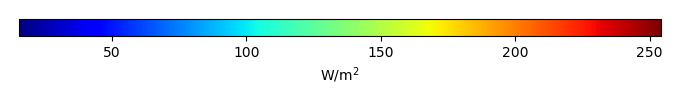MODEL MEANBIAS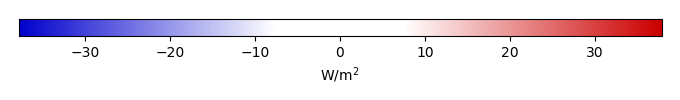BIAS SCORERMSE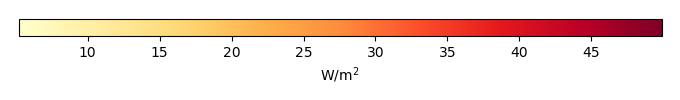RMSE SCOREBENCHMARK INTERANNUAL VARIABILITY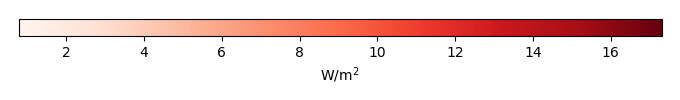MODEL INTERANNUAL VARIABILITYINTERANNUAL VARIABILITY SCOREBENCHMARK MAX MONTHMODEL MAX MONTHDIFFERENCE IN MAX MONTH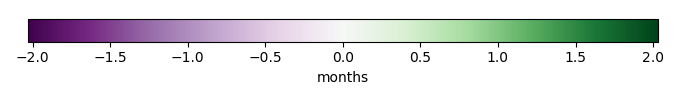SEASONAL CYCLE SCORESPATIAL TAYLOR DIAGRAMMODEL COLORS# Spatially integrated regional mean

MODEL COLORSREGIONAL MEANANNUAL CYCLEMONTHLY ANOMALYANNUAL CYCLE# All Models

BenchmarkCLM4CLM4.5CLM5# Data Information

creation_date: Thu May 8 23:03:44 PDT 2014

source_file: This product is generated from monthly 1 degree CERES EBAF Radiation observations